# Alternative Dice

Here is an fun proof I saw during a stay at MSRI (Mathematical Sciences Research Institute) – unfortunately, I can not remember the reference (or none was given). If you happen to know the originator of this work, please let me know and I will attribute it appropriately. In any event, it is a “fun” problem, so I typed up the following summary:

Prop: There exists precisely one pair of numerically non-symmetric six-sided dice with no blank sides such that the sum-roll probability distribution is equivalent to normal symmetric six-sided dice. (Where by sum-roll it is meant the probability of two dice rolling a sum of x, etc.)

Proof: Let a die be represented by a Polynomial in the following fashion. Powers represent the number on a side of a die, and the coefficient of a specific power represents the number of sides with that number of dots. For example, a normal symmetric die has the following representation:$P(x) = x + x^2 + x^3 + x^4 + x^5 + x^6$

And a four sided die with three sevens and one four would be:$P(x) = x^4 + 3*x^7$

Notice that with this representation,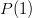$P(1)$ is the number of sides on the die. Also notice that this representation encapsulates the probability of a specific roll, i.e., the probability of a die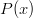$P(x)$ rolling a “n” is: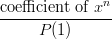$\dfrac{\text{coefficient of }x^n}{P(1)}$

Finally, the action of rolling two dice is equivalent to forming the product of the polynomial representations. For example, when two normal symmetric six-sided dice are rolled, the probability of rolling a one (in sum) is zero, the probability of rolling a two is 1/36, the probability of rolling a seven is 1/6, etc. Notice that if we form the product$(x + x^2 + x^3 + x^4 + x^5 + x^6)^2$

Then the coefficient of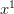$x^1$ is zero, the coefficient of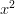$x^2$ is one, and the coefficient of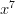$x^7$ is six (0/36, 1/36, and 6/36).

Now, to answer the original question, we find two polynomials,$T(x)$ and$S(x)$ such that:

1)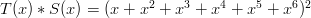$T(x)*S(x) = (x + x^2 + x^3 + x^4 + x^5 + x^6)^2$
2)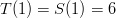$T(1) = S(1) = 6$
3)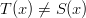$T(x) \neq S(x)$
4)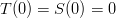$T(0) = S(0) = 0$

Condition #1 gives us the same sum-roll distribution as normal symmetric dice.
Condition #2 gives us two six-sided dice.
Condition #3 assures us of the non-symmetry of$T(x)$ and$S(x)$.
Condition #4 is equivalent to saying that the polynomials have a zero coefficient for the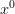$x^0$ term, which is the same as saying that the dice have no blank sides.

So, to proceed, we simply need to factor the polynomial in condition #1. Here it is:$x^2 * (x^2 + 1)^2 * (x^2 - x + 1)^2 * (x^2 + x + 1)^2$

Which I’ll write as: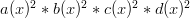$a(x)^2 * b(x)^2 * c(x)^2 * d(x)^2$

where: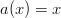$a(x) = x$$b(x) = x^2 + 1$$c(x) = x^2 - x + 1$$d(x) = x^2 + x + 1$

Notice that$a(1)=1$ so multiplying by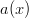$a(x)$ will not change the number of sides on the die. Also notice that if we put both$a(x)$ terms in one of$T(x)$ or$S(x)$ then the other die would have a blank side (since the product of any of the remaining terms$b(x)$,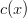$c(x)$ or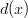$d(x)$ would incur a monomial). Thus, both$T(x)$ and$S(x)$ must have$a(x)$ as a factor.

Now, notice that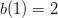$b(1)=2$ and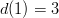$d(1)=3$, whose product is six. Also notice that$c(1)=1$. Thus, if we want$T(x)$ and$S(x)$ to represent six-sided dice then they must both have$b(x)$ and$d(x)$ as factors, since multiplying by$c(x)$ will not change the number of sides. So far we have
the following:$T(x) = a(x) * b(x) * d(x) * ??$$S(x) = a(x) * b(x) * d(x) * ??$

The only factor we have left is the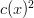$c(x)^2$. If we put a$c(x)$ in both of$T(x)$ and$S(x)$ then we will have created symmetric dice since$T(x)$ will be equal to$S(x)$ (in violation of condition #3). The only other option is to put the entire$c(x)^2$ term into either$T(x)$ or$S(x)$ By symmetry, it is irrelevant which polynomial gets the factor, so we’ll try:$T(x) = a(x) * b(x) * c(x)^2 * d(x)$$S(x) = a(x) * b(x) * d(x)$

Now let’s check our work! Condition #1 is satisfied since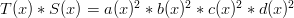$T(x)*S(x) = a(x)^2 * b(x)^2 * c(x)^2 * d(x)^2$. How about condition #2? Well,$T(1) = 6$ and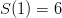$S(1) = 6$ so that looks good. Clearly,$T(x) != S(x)$ so condition #3 is ok. Finally, neither term has a monic, so$T(0)=S(0)=0$ and neither die has a blank side.

So, now you have all the information you need to find these dice. I didn’t want to spoil it by writing out the actual sides, so I’ll leave you the final step.

#### Alternative Dice — 1 Comment

1.Avery on said:

I saw this pair of dice around 2006. I don’t remember a proof, but stumbled across yours as I was inspired to try to find the dice today.

As stated, a(x)^2 * b(x)^2 * c(x)^2 * d(x)^2 would yield a 14th degree polynomial, which would imply that one could roll a 14 on a normal pair of dice.

It seems that your b(x) is incorrect. If b(x)=x+1, things work out for a normal pair of dice.

The error was easy to miss since when x=1, x+1 = x^2 +1 = 2.

The proof using polynomials is clever. Definitely a different way to look at things. Yay math!